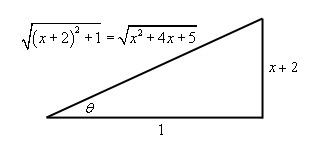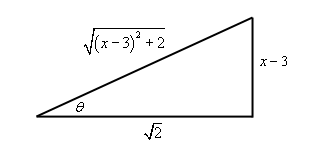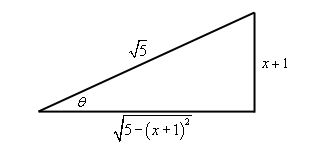Paul's Online Notes
Home / Calculus II / Integration Techniques / Integrals Involving Quadratics
Show Mobile Notice Show All Notes Hide All Notes
Mobile Notice
You appear to be on a device with a "narrow" screen width (i.e. you are probably on a mobile phone). Due to the nature of the mathematics on this site it is best views in landscape mode. If your device is not in landscape mode many of the equations will run off the side of your device (should be able to scroll to see them) and some of the menu items will be cut off due to the narrow screen width.

### Section 7.6 : Integrals Involving Quadratics

To this point we’ve seen quite a few integrals that involve quadratics. A couple of examples are,

$\int{{\frac{x}{{{x^2} \pm a}}\,dx}} = \frac{1}{2}\ln \left| {{x^2} \pm a} \right| + c\hspace{0.5in}\int{{\frac{1}{{{x^2} + {a^2}}}\,dx = \frac{1}{a}{{\tan }^{ - 1}}\left( {\frac{x}{a}} \right)+c}}$

We also saw that integrals involving $$\sqrt {{b^2}{x^2} - {a^2}}$$, $$\sqrt {{a^2} - {b^2}{x^2}}$$ and $$\sqrt {{a^2} + {b^2}{x^2}}$$ could be done with a trig substitution.

Notice however that all of these integrals were missing an $$x$$ term. They all consist of only a quadratic term and a constant.

Some integrals involving general quadratics are easy enough to do. For instance, the following integral can be done with a quick substitution.

\begin{align*}\int{{\frac{{2x + 3}}{{4{x^2} + 12x - 1}}\,dx}} & = \frac{1}{4}\int{{\frac{1}{u}\,du}}\hspace{0.5in}u = 4{x^2} + 12x - 1\hspace{0.25in}du = 4\left( {2x + 3} \right)dx\\ & = \frac{1}{4}\ln \left| {4{x^2} + 12x - 1} \right| + c\end{align*}

Some integrals with quadratics can be done with partial fractions. For instance,

$\int{{\frac{{10x - 6}}{{3{x^2} + 16x + 5}}\,dx}} = \int{{\frac{4}{{x + 5}} - \frac{2}{{3x + 1}}\,dx}} = 4\ln \left| {x + 5} \right| - \frac{2}{3}\ln \left| {3x + 1} \right| + c$

Unfortunately, these methods won’t work on a lot of integrals. A simple substitution will only work if the numerator is a constant multiple of the derivative of the denominator and partial fractions will only work if the denominator can be factored.

The topic of this section is how to deal with integrals involving quadratics when the techniques that we’ve looked at to this point simply won’t work.

Back in the Trig Substitution section we saw how to deal with square roots that had a general quadratic in them. Let’s take a quick look at another one like that since the idea involved in doing that kind of integral is exactly what we are going to need for the other integrals in this section.

Example 1 Evaluate the following integral. $\int{{\sqrt {{x^2} + 4x + 5} \,dx}}$
Show Solution

Recall from the Trig Substitution section that in order to do a trig substitution here we first needed to complete the square on the quadratic. This gives,

${x^2} + 4x + 5 = {x^2} + 4x + 4 - 4 + 5 = {\left( {x + 2} \right)^2} + 1$

After completing the square the integral becomes,

$\int{{\sqrt {{x^2} + 4x + 5} \,dx}} = \int{{\sqrt {{{\left( {x + 2} \right)}^2} + 1} \,dx}}$

Upon doing this we can identify the trig substitution that we need. Here it is,

$x + 2 = \tan \theta \hspace{0.5in}x = \tan \theta - 2\hspace{0.5in}dx = {\sec ^2}\theta \,d\theta$ $\sqrt {{{\left( {x + 2} \right)}^2} + 1} = \sqrt {{{\tan }^2}\theta + 1} = \sqrt {{{\sec }^2}\theta } = \left| {\sec \theta } \right| = \sec \theta$

Recall that since we are doing an indefinite integral we can drop the absolute value bars. Using this substitution the integral becomes,

\begin{align*}\int{{\sqrt {{x^2} + 4x + 5} \,dx}} & = \int{{{{\sec }^3}\theta \,d\theta }}\\ & = \frac{1}{2}\left( {\sec \theta \tan \theta + \ln \left| {\sec \theta + \tan \theta } \right|} \right) + c\end{align*}

We can finish the integral out with the following right triangle.

$\tan \theta = \frac{{x + 2}}{1}\hspace{0.5in}\sec \theta = \frac{{\sqrt {{x^2} + 4x + 5} }}{1} = \sqrt {{x^2} + 4x + 5}$$\int{{\sqrt {{x^2} + 4x + 5} \,dx}} = \frac{1}{2}\left( {\left( {x + 2} \right)\sqrt {{x^2} + 4x + 5} + \ln \left| {x + 2 + \sqrt {{x^2} + 4x + 5} } \right|} \right) + c$

So, by completing the square we were able to take an integral that had a general quadratic in it and convert it into a form that allowed us to use a known integration technique.

Let’s do a quick review of completing the square before proceeding. Here is the general completing the square formula that we’ll use.

${x^2} + bx + c = {x^2} + bx + {\left( {\frac{b}{2}} \right)^2} - {\left( {\frac{b}{2}} \right)^2} + c = {\left( {x + \frac{b}{2}} \right)^2} + c - \frac{{{b^2}}}{4}$

This will always take a general quadratic and write it in terms of a squared term and a constant term.

Recall as well that in order to do this we must have a coefficient of one in front of the $${x^2}$$. If not, we’ll need to factor out the coefficient before completing the square. In other words,

$a{x^2} + bx + c = a \;\; {\underbrace { \left( {x^2} + \frac{b}{a}x + \frac{c}{a}\right)}_{\scriptstyle{\mbox{complete the }}\atop\scriptstyle{\mbox{square on this!}}}}$

Now, let’s see how completing the square can be used to do integrals that we aren’t able to do at this point.

Example 2 Evaluate the following integral. $\int{{\frac{1}{{2{x^2} - 3x + 2}}\,dx}}$
Show Solution

Okay, this doesn’t factor so partial fractions just won’t work on this. Likewise, since the numerator is just “1” we can’t use the substitution $$u = 2{x^2} - 3x + 2$$. So, let’s see what happens if we complete the square on the denominator.

\begin{align*}2{x^2} - 3x + 2 & = 2\left( {{x^2} - \frac{3}{2}x + 1} \right)\\ & = 2\left( {{x^2} - \frac{3}{2}x + \frac{9}{{16}} - \frac{9}{{16}} + 1} \right)\\ & = 2\left( {{{\left( {x - \frac{3}{4}} \right)}^2} + \frac{7}{{16}}} \right)\end{align*}

With this the integral is,

$\int{{\frac{1}{{2{x^2} - 3x + 2}}\,dx}} = \frac{1}{2}\int{{\frac{1}{{{{\left( {x - \frac{3}{4}} \right)}^2} + \frac{7}{{16}}}}\,dx}}$

Now this may not seem like all that great of a change. However, notice that we can now use the following substitution.

$u = x - \frac{3}{4}\hspace{0.5in}du = dx$

and the integral is now,

$\int{{\frac{1}{{2{x^2} - 3x + 2}}\,dx}} = \frac{1}{2}\int{{\frac{1}{{{u^2} + \frac{7}{{16}}}}\,du}}$

We can now see that this is an inverse tangent! So, using the formula from the start of the section we get,

\begin{align*}\int{{\frac{1}{{2{x^2} - 3x + 2}}\,dx}} & = \frac{1}{2}\left( {\frac{4}{{\sqrt 7 }}} \right){\tan ^{ - 1}}\left( {\frac{{4u}}{{\sqrt 7 }}} \right) + c\\ & = \frac{2}{{\sqrt 7 }}{\tan ^{ - 1}}\left( {\frac{{4x - 3}}{{\sqrt 7 }}} \right) + c\end{align*}
Example 3 Evaluate the following integral. $\int{{\frac{{3x - 1}}{{{x^2} + 10x + 28}}\,dx}}$
Show Solution

This example is a little different from the previous one. In this case we do have an $$x$$ in the numerator however the numerator still isn’t a multiple of the derivative of the denominator and so a simple Calculus I substitution won’t work.

So, let’s again complete the square on the denominator and see what we get,

${x^2} + 10x + 28 = {x^2} + 10x + 25 - 25 + 28 = {\left( {x + 5} \right)^2} + 3$

Upon completing the square the integral becomes,

$\int{{\frac{{3x - 1}}{{{x^2} + 10x + 28}}\,dx}} = \int{{\frac{{3x - 1}}{{{{\left( {x + 5} \right)}^2} + 3}}\,dx}}$

At this point we can use the same type of substitution that we did in the previous example. The only real difference is that we’ll need to make sure that we plug the substitution back into the numerator as well.

$u = x + 5\hspace{0.5in}x = u - 5\hspace{0.5in}dx = du$ \begin{align*}\int{{\frac{{3x - 1}}{{{x^2} + 10x + 28}}\,dx}} & = \int{{\frac{{3\left( {u - 5} \right) - 1}}{{{u^2} + 3}}\,du}}\\ & = \int{{\frac{{3u}}{{{u^2} + 3}} - \frac{{16}}{{{u^2} + 3}}\,du}}\\ & = \frac{3}{2}\ln \left| {{u^2} + 3} \right| - \frac{{16}}{{\sqrt 3 }}{\tan ^{ - 1}}\left( {\frac{u}{{\sqrt 3 }}} \right) + c\\ & = \frac{3}{2}\ln \left| {{{\left( {x + 5} \right)}^2} + 3} \right| - \frac{{16}}{{\sqrt 3 }}{\tan ^{ - 1}}\left( {\frac{{x + 5}}{{\sqrt 3 }}} \right) + c\end{align*}

So, in general when dealing with an integral in the form,

$\begin{equation}\int{{\frac{{Ax + B}}{{a{x^2} + bx + c}}\,dx}} \label{eq:eq1} \end{equation}$

Here we are going to assume that the denominator doesn’t factor and the numerator isn’t a constant multiple of the derivative of the denominator. In these cases, we complete the square on the denominator and then do a substitution that will yield an inverse tangent and/or a logarithm depending on the exact form of the numerator.

Let’s now take a look at a couple of integrals that are in the same general form as $$\eqref{eq:eq1}$$ except the denominator will also be raised to a power. In other words, let’s look at integrals in the form,

$\begin{equation}\int{{\frac{{Ax + B}}{{{{\left( {a{x^2} + bx + c} \right)}^n}}}\,dx}} \label{eq:eq2} \end{equation}$
Example 4 Evaluate the following integral. $\int{{\frac{x}{{{{\left( {{x^2} - 6x + 11} \right)}^3}}}\,dx}}$
Show Solution

For the most part this integral will work the same as the previous two with one exception that will occur down the road. So, let’s start by completing the square on the quadratic in the denominator.

${x^2} - 6x + 11 = {x^2} - 6x + 9 - 9 + 11 = {\left( {x - 3} \right)^2} + 2$

The integral is then,

$\int{{\frac{x}{{{{\left( {{x^2} - 6x + 11} \right)}^3}}}\,dx}} = \int{{\frac{x}{{{{\left[ {{{\left( {x - 3} \right)}^2} + 2} \right]}^3}}}\,dx}}$

Now, we will use the same substitution that we’ve used to this point in the previous two examples.

$u = x - 3\hspace{0.5in}x = u + 3\hspace{0.5in}dx = du$ \begin{align*}\int{{\frac{x}{{{{\left( {{x^2} - 6x + 11} \right)}^3}}}\,dx}} & = \int{{\frac{{u + 3}}{{{{\left( {{u^2} + 2} \right)}^3}}}\,du}}\\ & = \int{{\frac{u}{{{{\left( {{u^2} + 2} \right)}^3}}}\,du}} + \int{{\frac{3}{{{{\left( {{u^2} + 2} \right)}^3}}}\,du}}\end{align*}

Now, here is where the differences start cropping up. The first integral can be done with the substitution $$v = {u^2} + 2$$ and isn’t too difficult. The second integral however, can’t be done with the substitution used on the first integral and it isn’t an inverse tangent.

It turns out that a trig substitution will work nicely on the second integral and it will be the same as we did when we had square roots in the problem.

$u = \sqrt 2 \tan \theta \hspace{0.5in}du = \sqrt 2 {\sec ^2}\theta \,d\theta$

With these two substitutions the integrals become,

\begin{align*}\int{{\frac{x}{{{{\left( {{x^2} - 6x + 11} \right)}^3}}}\,dx}} & = \frac{1}{2}\int{{\frac{1}{{{v^3}}}\,dv}} + \int{{\frac{3}{{{{\left( {2{{\tan }^2}\theta + 2} \right)}^3}}}\,\left( {\sqrt 2 {{\sec }^2}\theta } \right)d\theta }}\\ & = - \frac{1}{4}\frac{1}{{{v^2}}} + \int{{\frac{{3\sqrt 2 {{\sec }^2}\theta }}{{8{{\left( {{{\tan }^2}\theta + 1} \right)}^3}}}\,d\theta }}\\ & = - \frac{1}{4}\frac{1}{{{{\left( {{u^2} + 2} \right)}^2}}} + \frac{{3\sqrt 2 }}{8}\int{{\frac{{{{\sec }^2}\theta }}{{{{\left( {{{\sec }^2}\theta } \right)}^3}}}\,d\theta }}\\ & = - \frac{1}{4}\frac{1}{{{{\left( {{{\left( {x - 3} \right)}^2} + 2} \right)}^2}}} + \frac{{3\sqrt 2 }}{8}\int{{\frac{1}{{{{\sec }^4}\theta }}\,d\theta }}\\ & = - \frac{1}{4}\frac{1}{{{{\left( {{{\left( {x - 3} \right)}^2} + 2} \right)}^2}}} + \frac{{3\sqrt 2 }}{8}\int{{{{\cos }^4}\theta \,d\theta }}\end{align*}

Okay, at this point we’ve got two options for the remaining integral. We can either use the ideas we learned in the section about integrals involving trig integrals or we could use the following formula.

$\int{{{{\cos }^m}\theta \,d\theta }} = \frac{1}{m}\sin \theta {\cos ^{m - 1}}\theta + \frac{{m - 1}}{m}\int{{{{\cos }^{m - 2}}\theta \,d\theta }}$

Let’s use this formula to do the integral.

\begin{align*}\int{{{{\cos }^4}\theta \,d\theta }} & = \frac{1}{4}\sin \theta {\cos ^3}\theta + \frac{3}{4}\int{{{{\cos }^2}\theta \,d\theta }}\\ & = \frac{1}{4}\sin \theta {\cos ^3}\theta + \frac{3}{4}\left( {\frac{1}{2}\sin \theta \cos \theta + \frac{1}{2}\int{{{{\cos }^0}\theta \,d\theta }}} \right)\hspace{0.25in}{\cos ^0}\theta = 1!\\ & = \frac{1}{4}\sin \theta {\cos ^3}\theta + \frac{3}{8}\sin \theta \cos \theta + \frac{3}{8}\theta \end{align*}

Next, let’s use the following right triangle to get this back to $$x$$’s.

$\tan \theta = \frac{u}{{\sqrt 2 }} = \frac{{x - 3}}{{\sqrt 2 }}\hspace{0.5in}\sin \theta = \frac{{x - 3}}{{\sqrt {{{\left( {x - 3} \right)}^2} + 2} }}\hspace{0.5in}\cos \theta = \frac{{\sqrt 2 }}{{\sqrt {{{\left( {x - 3} \right)}^2} + 2} }}$The cosine integral is then,

\begin{align*}\int{{{{\cos }^4}\theta \,d\theta }} & = \frac{1}{4}\frac{{2\sqrt 2 \left( {x - 3} \right)}}{{{{\left( {{{\left( {x - 3} \right)}^2} + 2} \right)}^2}}} + \frac{3}{8}\frac{{\sqrt 2 \left( {x - 3} \right)}}{{{{\left( {x - 3} \right)}^2} + 2}} + \frac{3}{8}{\tan ^{ - 1}}\left( {\frac{{x - 3}}{{\sqrt 2 }}} \right)\\ & = \frac{{\sqrt 2 }}{2}\frac{{x - 3}}{{{{\left( {{{\left( {x - 3} \right)}^2} + 2} \right)}^2}}} + \frac{{3\sqrt 2 }}{8}\frac{{x - 3}}{{{{\left( {x - 3} \right)}^2} + 2}} + \frac{3}{8}{\tan ^{ - 1}}\left( {\frac{{x - 3}}{{\sqrt 2 }}} \right)\end{align*}

All told then the original integral is,

\begin{align*}\int{{\frac{x}{{{{\left( {{x^2} - 6x + 11} \right)}^3}}}\,dx}} & = - \frac{1}{4}\frac{1}{{{{\left( {{{\left( {x - 3} \right)}^2} + 2} \right)}^2}}} + \\ & \hspace{1.0in}\frac{{3\sqrt 2 }}{8}\left( {\frac{{\sqrt 2 }}{2}\frac{{x - 3}}{{{{\left( {{{\left( {x - 3} \right)}^2} + 2} \right)}^2}}} + \frac{{3\sqrt 2 }}{8}\frac{{x - 3}}{{{{\left( {x - 3} \right)}^2} + 2}} + \frac{3}{8}{{\tan }^{ - 1}}\left( {\frac{{x - 3}}{{\sqrt 2 }}} \right)} \right)\\ & \\ & = \frac{1}{8}\frac{{3x - 11}}{{{{\left( {{{\left( {x - 3} \right)}^2} + 2} \right)}^2}}} + \frac{9}{{32}}\frac{{x - 3}}{{{{\left( {x - 3} \right)}^2} + 2}} + \frac{{9\sqrt 2 }}{{64}}{\tan ^{ - 1}}\left( {\frac{{x - 3}}{{\sqrt 2 }}} \right) + c\end{align*}

It’s a long and messy answer, but there it is.

Example 5 Evaluate the following integral. $\int{{\frac{{x - 3}}{{{{\left( {4 - 2x - {x^2}} \right)}^2}}}\,dx}}$
Show Solution

As with the other problems we’ll first complete the square on the denominator.

$4 - 2x - {x^2} = - \left( {{x^2} + 2x - 4} \right) = - \left( {{x^2} + 2x + 1 - 1 - 4} \right) = - \left( {{{\left( {x + 1} \right)}^2} - 5} \right) = 5 - {\left( {x + 1} \right)^2}$

The integral is,

$\int{{\frac{{x - 3}}{{{{\left( {4 - 2x - {x^2}} \right)}^2}}}\,dx}} = \int{{\frac{{x - 3}}{{{{\left[ {5 - {{\left( {x + 1} \right)}^2}} \right]}^2}}}\,dx}}$

Now, let’s do the substitution.

$u = x + 1\hspace{0.5in}x = u - 1\hspace{0.5in}dx = du$

and the integral is now,

\begin{align*}\int{{\frac{{x - 3}}{{{{\left( {4 - 2x - {x^2}} \right)}^2}}}\,dx}} & = \int{{\frac{{u - 4}}{{{{\left( {5 - {u^2}} \right)}^2}}}\,du}}\\ & = \int{{\frac{u}{{{{\left( {5 - {u^2}} \right)}^2}}}\,du}} - \int{{\frac{4}{{{{\left( {5 - {u^2}} \right)}^2}}}\,du}}\end{align*}

In the first integral we’ll use the substitution

$v = 5 - {u^2}$

and in the second integral we’ll use the following trig substitution

$u = \sqrt 5 \sin \theta \hspace{0.5in}du = \sqrt 5 \cos \theta \,d\theta$

Using these substitutions the integral becomes,

\begin{align*}\int{{\frac{{x - 3}}{{{{\left( {4 - 2x - {x^2}} \right)}^2}}}\,dx}} & = - \frac{1}{2}\int{{\frac{1}{{{v^2}}}\,dv}} - \int{{\frac{4}{{{{\left( {5 - 5{{\sin }^2}\theta } \right)}^2}}}\left( {\sqrt 5 \cos \theta } \right)\,d\theta }}\\ & = \frac{1}{2}\frac{1}{v} - \frac{{4\sqrt 5 }}{{25}}\int{{\frac{{\cos \theta }}{{{{\left( {1 - {{\sin }^2}\theta } \right)}^2}}}\,d\theta }}\\ & = \frac{1}{2}\frac{1}{v} - \frac{{4\sqrt 5 }}{{25}}\int{{\frac{{\cos \theta }}{{{{\cos }^4}\theta }}\,d\theta }}\\ & = \frac{1}{2}\frac{1}{v} - \frac{{4\sqrt 5 }}{{25}}\int{{{{\sec }^3}\theta \,d\theta }}\\ & = \frac{1}{2}\frac{1}{v} - \frac{{2\sqrt 5 }}{{25}}\left( {\sec \theta \tan \theta + \ln \left| {\sec \theta + \tan \theta } \right|} \right) + c\end{align*}

We’ll need the following right triangle to finish this integral out.

$\sin \theta = \frac{u}{{\sqrt 5 }} = \frac{{x + 1}}{{\sqrt 5 }}\hspace{0.5in}\sec \theta = \frac{{\sqrt 5 }}{{\sqrt {5 - {{\left( {x + 1} \right)}^2}} }}\hspace{0.5in}\tan \theta = \frac{{x + 1}}{{\sqrt {5 - {{\left( {x + 1} \right)}^2}} }}$So, going back to $$x$$’s the integral becomes,

\begin{align*}\int{{\frac{{x - 3}}{{{{\left( {4 - 2x - {x^2}} \right)}^2}}}\,dx}} & = \frac{1}{2}\frac{1}{{5 - {u^2}}} - \frac{{2\sqrt 5 }}{{25}}\left( {\frac{{\sqrt 5 \left( {x + 1} \right)}}{{5 - {{\left( {x + 1} \right)}^2}}} + \ln \left| {\frac{{\sqrt 5 }}{{\sqrt {5 - {{\left( {x + 1} \right)}^2}} }} + \frac{{x + 1}}{{\sqrt {5 - {{\left( {x + 1} \right)}^2}} }}} \right|} \right) + c\\ & = \frac{1}{{10}}\frac{{1 - 4x}}{{5 - {{\left( {x + 1} \right)}^2}}} - \frac{{2\sqrt 5 }}{{25}}\ln \left| {\frac{{x + 1 + \sqrt 5 }}{{\sqrt {5 - {{\left( {x + 1} \right)}^2}} }}} \right| + c\end{align*}

Often the following formula is needed when using the trig substitution that we used in the previous example.

$\int{{{{\sec }^m}\theta \,d\theta }} = \frac{1}{{m - 1}}\tan \theta {\sec ^{m - 2}}\theta + \frac{{m - 2}}{{m - 1}}\int{{{{\sec }^{m - 2}}\theta \,d\theta }}$

Note that we’ll only need the two trig substitutions (sine and tangent) that we used here. The third trig substitution (secant) that we used will not be needed here. Any quadratic that could use a secant substitution can be turned into a sine substitution simply by factoring a minus sign out of the quadratic. Note that we can do that for these types of problems because we don’t have a root and so the minus sign can be completely factored out of the integrand while we couldn’t do that with the roots we had in the problems back in the Trig Substitution section.